Rubik's Cube Solutions plus Puzzles and 8-Balls

Contents:
Introduction #intro
Basic Cube Group Theory #basiccubetheory
Rubik's Cube Solution #solution
Cube Solution Supplement for Patterned Faces #patternsupplement
Rubik's Revenge Solution #revengesolution
Advanced Cube Group Theory #grouptheory
My Cube and Puzzle Collection #cubefamily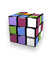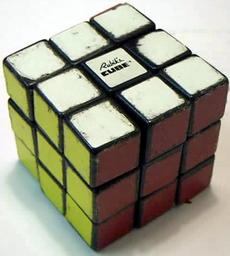My old racing Rubik's Cube http://www.rubiks.com is well-worn and barely holds together. I gave it a lot of wear in June 1982 during the week between high school finals and graduation ../../scscards/liberty.html. A cube has 43,252,003,274,489,856,000 (over 4 X 10^19) different positions http://www-gap.dcs.st-and.ac.uk/~gap/Intro/rubik.html. This number is the product of 8! corner position permutations X 12!/2 edge position permutations X 3^8/3 corner twirls X 2^12/2 edge flips and factors as 2^27 X 3^14 X 5^3 X 7^2 X 11. If you take a cube apart, there are 12 different ways that you can put it together such no set of moves will take the cube from one of the 12 assemblies to the other. When I was in practice, I could solve a scrambled cube in about a minute using about 65 moves (counting slices as a single move). I usually do the top corners, three of the top edges, the bottom corners, the bottom edges, the last top edge, and then the center edges.A Rubik's Revenge Cube http://www.rubiks.com from 1982. A Rubik's Revenge Cube has 7,401,196,841,564,901,869,874,093,974,498,574,336,000,000,000 (over 7 X 10^45) different positions http://www.geocities.com/jaapsch/puzzles/cube4.htm. When I was in practice, it took about twice as long and about twice as many moves to solve as the original cube.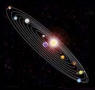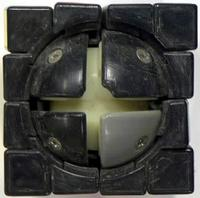A Rubik's Revenge Cube has a round center.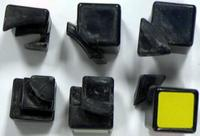Rubik's Revenge parts are fragile, especially the center pieces (on the left). The two pieces in the middle are edges, and the two pieces on the right are corners.

Version of this page in Belorussian http://onlinecasinospotlight.com/web/rubiks-cube-solutions-be
Jessica Fridrich's 17 second 56 move solution http://www.ws.binghamton.edu/fridrich/
Dan Knight's 17.3 second solution http://www.knightslab.org/#!speedcubing/cy62
Lars Petrus's 24 second 60 move solution http://lar5.com/cube/
Lego Robot Solves Rubik's Cube Puzzle In 3.253 Seconds http://tech.slashdot.org/story/14/03/19/0333218/lego-robot-solves-rubiks-cube-puzzle-in-3253-seconds
How to build a LEGO Speedcuber in under 5 minutes using ARM Powered devices https://www.youtube.com/watch?v=u7h5KSOFXSc
Robot solves cube in 12 seconds http://singularityhub.com/2010/02/17/lego-robot-solves-any-rubiks-cube-in-less-than-12-seconds-video/
Robot solves larger cubes http://singularityhub.com/2010/04/28/lego-robot-solves-bigger-and-harder-rubiks-cubes-video/
Android phone solves Rubik's cube in 12.5 seconds http://deviceguru.com/android-phone-controlled-robot-solves-rubiks-cube/
Philip Marshall's 65 move solution http://helm.lu/cube/MarshallPhilipp/
Christophe Goudey's CubeLand with solutions, games, contests and links http://cubeland.free.fr/
Michael Reid's links, patterns, and optimal solver http://www.cflmath.com/Rubik/index.html
Morley Davidson, John Dethridge, Herbert Kociemba, and Tomas Rokicki prove that the best solution is 20 moves http://www.cube20.org/
Herbert Kociemba's CubeExplorer that finds solutions of 19 moves on average http://kociemba.org/cube.htm
Cooperman and Kunkle's 26 move solution (May 2007) http://www.ddj.com/blog/portal/archives/2007/05/rubiks_cube_bus.html and http://www.sciencedaily.com/releases/2007/05/070531131326.htm and http://www.yubanet.com/artman/publish/article_58110.shtml
Tomas Rokicki's 25 move solution (March 2008) http://arxiv.org/abs/0803.3435 http://arxivblog.com/?p=332
Demaine, Demaine, Eisenstat, Lubiw, and Winslow's Algorithms for Solving Rubik's Cubes (June 2011) http://arxiv.org/abs/1106.5736
Matthew Monroe's page http://jjorg.chem.unc.edu/personal/monroe/cube/rubik.html
Josef "Gloom" Jelinek's and Dr. Hana M. Bizek's solution page http://cube.misto.cz/
Mark Jeays' solution page http://jeays.net/rubiks.htm
Diego de Pereda's solution page http://thecube.guru/rubiks-cube-solution/
R. A. Hoffman's graphic solution http://www.mts.net/~rhoffman/index.htm
Peter's Cube Page http://www.speedcubing.com/peter/
Chris Hardwick's Cube Page http://www.speedcubing.com/chris/
Cubeman's page http://cubeman.vg-network.com/ http://cubeman.org/
Ryan Heise's Cube Page http://www.ryanheise.com/cube/
PuzzleSolver.com http://www.puzzlesolver.com/
Solution Guide for Beginners http://www.rubiksplace.com/
Jim Cranwell's NxNxN cube solution page http://www.geocities.com/xulfrepus/rubik/cube.html
4D cube http://www.superliminal.com/cube/cube.htm
Werner Randelshofer's disassembled cube and pattern collection http://www.randelshofer.ch/
Joshua's Virtual Puzzle Museum http://www.twistypuzzles.com/
W. D. Joyner's cube theory lecture notes http://web.usna.navy.mil/~wdj/rubik_nts.htm
Jim Mahoney's Group Theory & Rubik's Cube lecture notes http://www.marlboro.edu/~mahoney/courses/Spr00/rubik.html
Speed Cubing page http://www.speedcubing.com/
Gilles and his cubes http://grrroux.free.fr/
Jasmine's Speed Cuber Blog http://speedcuber.blogspot.com/
Georges Helm's page with a 600-item bibliography http://webplaza.pt.lu/geohelm/myweb/cubeold.htm
Official Rubik's Cube web site http://www.rubikscube.com/
Meffert's Puzzles sells various type of cubes and puzzles http://www.mefferts.com/
Magic Cube 4D http://www.superliminal.com/cube/cube.htm
Wikipedia has a cube overview http://en.wikipedia.org/wiki/Rubik%27s_Cube and optimal solutions http://en.wikipedia.org/wiki/Optimal_solutions_for_Rubik%27s_Cube
Yahoo Speed Solving Group http://games.groups.yahoo.com/group/speedsolvingrubikscube/
Discussions on the mathematics of the cube http://cubezzz.homelinux.org/drupal/
The most complicated yet elegant artifact http://shl.stanford.edu:3455/TenThings/417

Some basic cube theory:
A 3x3x3 cube has 27 pieces: 8 corners with three external sides, 12 edges with two external sides, 6 centers with one external side, and the middle with no external sides.
Cube moves work differently than arithmetic. On a calculator, if you add 1, then add 2, then subtract 1, and then subtract 2, you go back to 0 where you started. On a solved cube, if you make a move, then make a second move, then do the first move in reverse, and then do the second move in reverse, the cube will usually end up messed up. Order matters on a cube so that 1 plus 2 is often not the same as 2 plus 1. In group theory terms, two elements commute if they produce the same result when applied in either order. A group is Abelian if every pair of elements in the group commutes. The group formed by addition on the set of integers is Abelian, while the group formed by moves on a cube is non-Abelian.
What helps in finding solutions is that 1 plus 2 is often close enough to 2 plus 1 that the result of 1 plus 2 minus 1 minus 2 leaves only a few pieces out of place. Sequences of moves that leave only a few pieces out of places are building blocks for solving the cube. In group theory, moves of the format X Y X-in-reverse Y-in-reverse are called commutators. I will use the non-standard notation X * Y for X Y X' Y'. The usual group theory notation is (X,Y) = X' Y' X Y.
If you repeat any sequence of moves, you will eventually return to your starting position. Any move affects only a fixed number of pieces, and those pieces have a fixed number of different permutations. Once you repeat the move more times than the number of permutations, you must return to a permutation that you saw before. Repeating a sequence of moves often produces cube patterns with only a few cubes out of place, especially at repeat counts that evenly divide the number of times that you can repeat the move before returning to the initial position. These sequences are not good for fast solutions because they can be long and because there are often more direct ways to reach the positions, but they are good for exploring when you are starting out. In group theory, the sequence of moves that you repeated is a generator for the subgroup of the moves reachable by repeating that sequence of moves. The subgroup is also called the orbit of the element that you used as the generator. The number of times that you can repeat the move until you reach the starting position is called the order of the subgroup.
Once you find a move sequence that shifts a piece from one position to another position, you can use it to shift other pieces. You first do a setup move to place the piece that you want to move into the position that your move touches, then you do your move, and then you undo the setup move. It does not matter how much your setup move or your shifting move scrambles the cube, except that your setup move should not leave any solved pieces in positions that your shifting move will scramble. Moves of the format X Y X-in-reverse are called conjugate moves. I will use the non-standard notation X + Y for X Y X'.
To continue to more advanced cube group theory, see #grouptheory.
For an introduction to cube group theory, see http://members.tripod.com/~dogschool/.
For some basic hints, see http://remus.rutgers.edu/~rhoads/Fun/Rubik.hints.

When you are starting, if you are lost, it is OK to start over by taking the cube apart as long as you are careful, but keep in mind that each time you take the cube apart, it will get a little looser until it no longer stays together. If you feel the need to play a trick on someone, you can put a cube together in the wrong way if you must, but never peel off a sticker -- they are glued on and not sticky underneath.
No one has yet found the shortest solution, but it is probably in the low 20's of moves. This means in reverse, that it takes at least 20 moves to mess up a cube completely. When someone who hasn't played with cubes much messes up a cube for me to solve, they often do not make enough moves, or enough of the right moves, to scramble the cube completely. If you are careful when solving the first face, some of other faces will sometimes solve themselves.
The original cube had the pairs of opposite faces as white and blue, green and yellow, and red and orange. You should note the layout of your cube before trying to solve it.
There is no magic secret to solving the cube quickly. Whatever system you use, the more moves that you memorize, the faster you can work. The fastest systems require memorizing several hundred moves, plus their inverses and reflections.
You also need fast fingers. A friend in high school who could solve cubes in about 24 seconds had a move where he could flick the right and upper faces in a single motion, although he would regularly explode his cube.
Avoid cubes with hollowed-out corner pieces. The edges around the holes can catch on other pieces as you make moves. Better cubes have smooth corners without large mold marks. You can sometimes file down excessive mold marks.
I have never found a good cube lubricant. Avoid petroleum products -- they dissolve and soften plastic. Graphite powder works, but it leaves messy black dust, does not last long, and makes a gummy goo if it mixes with any liquid lubricant. Some people say that silicone-based lubricants work well. The cube itself makes a fine dust of plastic powder as the pieces rub against each other when you use the cube. You can see some cube dust on the picture of the broken Rubik's Revenge Cube above. This cube dust acts as a lubricant when it is dry, but it can get gummy if it mixes with a liquid lubricant.

My Rubik's Cube moves:
My notation is the standard U/D/R/L/F/B for a clockwise quarter turn of the outer slice of the face, U', etc., for a counter-clockwise quarter turn, and Us, etc., for a clockwise quarter turn of the middle slice with respect to the face.
An anti-slice is combination move of a face and the face opposite to it both clockwise or both counter-clockwise. The anti-slice Ua is UD, Ra is RL, Fa is FB, etc.
Read the moves from left to right, so that X Y means to do X and then Y. I sometimes add parentheses or spaces for readability or to help with memorization. The parentheses and spaces do not correspond to moves.
I also use some additional notation:
X * Y = X Y X' Y'
X + Y = X Y X'
X ^ 2 = X2 = X X
X ^ 3 = X3 = X X X
X ^ 4 = X4 = X X X X
X ^ n = repeat X an arbitrary number of times
This more compact notation shows the beauty and simplicity of the moves. It also helps to show how the moves work.
Repeat counts and inverses (') bind tighter than * and + so (X+Y') = (X+(Y')) = X Y' X'.
I sometimes temporarily shift the position of the cube in my hands as I make moves, especially for moves containing Fs. Some people have a notation for this (for example, x, y, and z for clockwise rotation from R, U, and F), but adding the shifting notation would make the move sequences longer without helping people who hold the cube differently than I hold it.
If you actually read these moves and find a mistake, please let me know. My email address is at the bottom of my home page ../../index.html.
Getting started:
I do the top corners and three of the four top edges first. I do not spend too much time deciding the first face to solve unless I am counting moves. To avoid wasting time, I usually pick the white or blue face, whichever is closest to the top when I first pick up the cube, unless I happen to notice that one face happens to have two or more correct pieces than the face that I would have picked.
Most of the web sites listed above have detailed instructions for solving the top layer.
Top corners:
Hold the cube so that the upper face has an unsolved corner in the UFR position. Rotate the bottom face so that the corner that belongs at UFR is at DFR. Find the position of the color of the upper face on the corner at DFR.
If it is at D, use R'D2R DR'D'R = (R'+D2)(D*R').
If it is at F, use FDF' = F+D.
If it is at R, use R'D'R = R'+D'.
If all of the remaining corners for the upper face are on the upper face, but in the wrong position, use one of these moves to shift one of the incorrect corners from the upper face to the bottom face. When you shift the corner to the bottom, try to avoid leaving the side with the color of the upper face on the bottom face.
You can sometimes place the first few corners in a single move with R or R2, etc., if the adjacent edges and corners are not yet positioned.
Top edges:
Hold the cube so that the upper face has the unsolved edge in the UF position.
If the edge that belongs at UF is on the middle slice (between the upper and bottom faces), rotate the middle slice to place the edge in the FR position and find the position of the color of the upper face on the FR edge.
If it is at F, use UL'Us2L = U(L'+Us2).
If it is at R, use ULUsL' = U(L+Us).
If the edge that belongs at UF is on the bottom face, rotate the bottom face to place the edge in the F position and find the position of the color of the upper face on the FD edge.
If it is at F, use D'Rs'DRs = D'*Rs'.
If it is at D, use Rs' D2 Rs = Rs'+D2.
If the edge that belongs at UF is on the upper face in the UF position with the color of the upper face on F, use F'UsF2Us2F' = (F'+Us)(F+Us2).
If the edge that belongs at UF is on the upper face in the UB position with the color of the upper face on U, use Rs2 D2 Rs2 = Rs2+D2.
If all of the remaining edges for the upper face are on the upper face, but in the wrong position, move the edge that belongs in UF to RB or LB with R if it is at UR, B or B' if it is at UB, or L' if it is at UL. Then use F or F', depending on the alignment of the edge, and then Us, Us2, or Us', depending on the position of the edge, to slide it into the slot on the F face, and then restore F and the other face.
Remember that you need to place only three of the top four edges. The fourth edge will be done in a later step.
Positioning the corners:
Next I turn the cube over, so that the almost-solved face is at the bottom and the unsolved face is at the top. Check the positions of the corners. You should be able to turn the top face so that either all of the corners are in place, only the two corners at the back are exchanged, or both the two corners at the front and the two corners at the back are exchanged.
If the two corners in the back are exchanged (4 in 6 chance), use FU'B'UF'U'B U2.
If both pairs of corners are exchanged (1 in 6 chance), use F URU'R' F' = F+(U*R).
Orienting the corners:
Next check the orientation of the corners. Either all of the corners will be correct, or two corners will be twirled with one corner clockwise and the other corner counter-clockwise, or three corners will be twirled either all clockwise or all counter-clockwise, or all four corners will be twirled with two corners clockwise and the other two corners counter-clockwise.
If only two corners are not oriented correctly (12 in 27 chance), rotate the upper face so that a bad corner is in the FR position and the color of its F face should be on the top. Use the move R'DR FDF' then rotate the upper face so that the second corner is in the FR position and finish with the move FD'F' R'D'R. The second half is the inverse of the first half. The entire move is ((R'+D)(F+D))*(U^n).
If three corners are not oriented correctly (8 in 27 chance), place the correctly oriented corner in the FL position and look at the corner in the FR position.
If the R face of the FR corner has the color that should be on top, use the move U2 F'U'FU'F' U2 F.
If the F face of the FR corner has the color that should be on top, use the move F' U2 FUF'UF U2. This is just the previous move in reverse.
If all four corners are not oriented correctly, rotate the top face so that the F face of the FL corner and the B face of the BL corner have the color that should go on top. Then look at the FR corner.
If the R face of the FR corner has the color that should be on top (4 in 27 chance), use the move F URU'R' URU'R' F' = F+((U*R)^2).
If the F face of the FR corner has the color that should be on top (2 in 27 chance), use the move R2U2 R U2R2 U = (R2U2+R)U.
If any other combinations appear, someone took your cube apart and did not put it back together correctly. You will need to pop it open to orient the corners.
Positioning the top and bottom edges:
Orient the cube so that the first face with all but one solved edge is at the left and the second face with only the four solved corners is at the right. From here on, the middle slice refers to the slice between the left and right faces.
Find an edge in the middle slice that belongs on the right face. Turn the middle slice so that the edge is on the bottom face, turn the left face so that the single unsolved edge is at the top, and turn the right face so that the position where the edge on the middle slice belongs is at the top. Turn the upper face a quarter-turn clockwise or counter-clockwise, depending on the orientation of the edge, rotate the middle slice to place the edge in position, and then turn the upper face back.
If the remaining edges for the right face are all in incorrect positions on the right face, rotate the right face to place one of the incorrect edges at the top and then use U Rs' U' to push it into the middle slice (or U Rs U' to push it into the left face if it has the correct orientation).
Very rarely, if all of the R face edges are on the R face but in incorrect positions, you can temporarily turn the cube to make the R face into the U face and use moves as if you were solving the cube top-middle-bottom. You can do a three-face cycle with F2 D (R2 Fs U2 Fs') D' F2 (cycles UF to UR, UR to UL, UL to UF), a three-face cycle with a flip with Rs' D (R2 Fs U2 Fs') D' Rs (same cycle but flips UR and UF), an adjacent edge-pair exchange with Fs2 D' (F2 Rs2 F2 Rs2) D Fs2 (exchanges UF with UR, UL with UB), an opposite edge-pair exchange with Fs2 D Rs2 D2 Rs2 D Fs2 (exchanges UF with UB, UL with UR), etc.
To place the last edge in the left face, instead of using a middle slice, use a three-edge cycle like F2 Rs F2 Rs' to avoid disturbing the right face. For example, to place DF in UL, use U' F2 Rs F2 Rs' U = U'+(F2*Rs).
If the last edge of the left face ends up in the last position of the right edge, you can place both edges at once if you line up the middle slice so that you need only a quarter turn. It is probably faster to do this even when one of the edges ends up with the wrong orientation. You can fix it later.
If the last left edge ends up in the last right position, and the last right edge ends up in the last left position, you can exchange them with U Rs2 U2 Rs2 U = U+(Rs2*U2).
Positioning the middle slice edges:
Now, only the middle slice is left. Orient the cube so that the two solved faces are at the left and right.
Place the edges in the correct position.
If three edges are out of position (8 in 12 chance), hold the cube so that the FD edge belongs in UB, the UB edge belongs in UF, and the UF edge belongs in FD, and then use F2 Rs' F2 Rs = F2*Rs'.
If four edges are out of position (3 in 12 chance), hold the cube so that UF and UB need to be exchanged, and DF and DB need to be exchanged, and then use D2 Rs2 D2 Rs2 = D2*Rs2.
If two edges are out of position, someone took your cube apart and put it together incorrectly. You might as well pop the cube apart now.
If one edge is out of position, someone exchanged a piece with another cube. You will need to find another cube and swap pieces.
Orienting the middle slice edges:
Now, either the cube will solved, or two edges will be flipped, or all four slice edges will be flipped.
If only two edges are flipped (6 in 8 chance), temporarily reorient the cube so that the solved faces are at the front and back and one of the flipped edges is at UR and the other is at UL, then use the move R'UsR2Us2R' U2 RUs2R2Us'R U2 = ((R'+Us)(R+Us2))*(U2). If the second flipped edge is at DL instead of UL, use L2 before and after the move.
If all four edges are flipped (1 in 8 chance), use the move F'L'F (RsU RsU RsU RsU) F'LF = (F'+L)+((RsU)^4).
If only one edge is flipped, someone has messed with your cube, and you will need to take your cube apart to fix it.
When I am solving the final edge of the top face, if it happens to be in position but flipped, I leave it and then orient it in this step along with the middle slice. If I end up with three flipped edges in the middle slice, I place the flipped edge from the previous step at UL, and I rotate the middle slice so that the single correctly flipped edge is at UF, and then I use the move RsU RsU RsU RsU.
Faster solutions:
One of the faster methods is to solve the top edges, then the top corners and middle edges at the same time, and then the bottom. The moves to position a top corner in combination with the middle edge below it are not much more complicated than just positioning the top corner. Finishing the bottom requires four steps: positioning and orienting the edges and positioning and orienting the corners. Faster solutions pair these into two steps that either position and then orient everything at once or that solve the edges and then solve the corners at once. In the easier, but less efficient, procedure that I use, my moves to position the bottom corners also twist them, and most of my moves for positioning and orienting corners also move the edges. You can take advantage of this by tracking the other pieces that the moves affect and coming up with similar moves for all of the cases.

Centers on Rubik's Cubes with patterned faces:
To solve a cube with pictures on the faces, first solve the cube without worrying about the pictures. After you follow the solution for a plain cube, everything should be in place, except that some centers might be rotated.
Find two centers that need opposite rotations. If all of the centers are rotated in the same direction, pick any two centers. Make the pattern called "dots" or "boxes" to place one center inside the face of the other center, then rotate that face to solve the center (if the center was rotated clockwise initially, rotate the face counter-clockwise), then undo the "dots" and unrotate the face. I usually start by placing the face with the center under consideration at the front of the cube and make "dots" by slicing that center through the top position.
Use 6 dots for adjacent centers on F and U, (Rs Bs Ls Fs) U^n (Fs' Ls' Bs' Rs') U^n' = (Rs Bs Ls Fs)*(U^n).
Use 4 dots for opposite centers on F and B, (Rs Bs2 Ls Fs2) B^n (Fs2 Ls' Bs2 Rs') B^n' = (Rs Bs2 Ls Fs2)*(B^n).
After repeating this procedure, you will either solve the cube or end up with only one rotated center. The rotated center will always be upside down. To solve it, hold the cube so that the face with the rotated center is on the top, and then make the move (RL U2 R'L') U (RL U2 R'L') U = ((Ra+U2)U)^2 (where U/R/L means rotating the upper/right/left face one-quarter turn clockwise, U2 means rotating the upper face one-half turn, R' means rotating the right face counter-clockwise, and Ra means the right anti-slice RL).

My Rubik's Revenge moves:
My notation is the standard U/D/R/L/F/B for a clockwise quarter turn of the outer slice of the face, Us, etc., for a clockwise quarter turn of the inner slice of the face, and U', etc., for a counter-clockwise quarter turn.
Note that slices on opposite faces like Us and Ds' refer to the same slice on a Rubik's Cube but to different slices on a Rubik's Revenge.
Some people use u for Us, etc., or U3 or U-1 or U- for U', etc.
I sometimes add parentheses or spaces for readability or to help with memorization. The parentheses and spaces do not correspond to moves.
Be careful moving the cube. When I try to go fast, I often end up jamming the cube or knocking out a piece. The movements are in my fingers, not in my head, and require no conscious thought. When the cube jams, it is hard to restart a sequence of moves from the middle.
After each move, I square-off the cube to keep the faces in alignment. This helps to keep the cube from jamming.
To reassemble an exploded cube, first do the centers, then build out from a corner, and do the final edges last. The centers have a thin leg that is easy to break. To pop in the last edge, it helps to turn the face 45 degrees. I have gone through a few different Rubik's Revenge cubes. The centers often break on the cubes with stiffer plastic, while the cubes with softer plastic often become too loose and fall apart.
I do the same top, bottom, middle routine as on a 3x3 cube, except that I also do the top and bottom centers along with the top and bottom corners and edges.
Unlike a 3x3 cube, you can not flip edges. The position of an edge determines its orientation. If you take a cube apart, you can see that the edges are rounded so that it is physically not possible to pop out a single edge, flip it upside down, and reinsert it. If an edge appears to be flipped, what really happened is that you are looking at the edge's pair that has the same colors but the opposite orientation. As a side note, unlike a 3x3 cube, it is possible to exchange any two edges. (In fact, I have the move sequence below as part of the solution.) If you exchange two adjacent edges, you can make a position that resembles the impossible single flipped edge on a 3x3 cube.
Top and bottom centers:
I first do the top and bottom centers. You can push the first few centers into position easily if you do two adjacent top centers, then do the two adjacent bottom centers across from the top centers (for example, if the top centers are at LF and LB, do the bottom centers at RF and RB), then turn the top or bottom face a half-turn, and do two more centers. Usually some centers are on diagonals instead of adjacent, and you won't get lucky enough to place all of the centers easily.
For the last few centers, find a center on the middle slice (i.e. Us or Ds) and a hole on the U face where the center belongs. Rotate Us or Ds to bring the center onto the F face, and then rotate the F face to bring the center to LD. Rotate the U face to bring the hole to RB. Then position the center with Rs' F' Rs.
You can reduce the number of setup moves by using reflections and inverses of this move:
(The center is always on the F face, and the hole is always on the U face.)
If the center is at LD, place the hole at RB, and use Rs' F' Rs.
If the center is at LU, place the hole at RF, and use Rs' F Rs.
If the center is at RU, place the hole at LF, and use Ls F' Ls'.
If the center is at RD, place the hole at LB, and use Ls F Ls'.
If two centers are at LU and LD, and you can place two holes at RF and RB, use Rs' F2 Rs.
If two centers are at RU and RD, and you can place two holes at LF and LB, use Ls F2 Ls'.
After you finish the top center, or if you ran out of top center pieces because some centers are on the bottom, flip the cube to bring the bottom to the top and use the same procedure for positioning centers.
You should be one with your cube and know all of the pairs of opposite colors so that you can place the correct color on the bottom. If you are not sure, check the colors on the corners. If you do the corners first, the centers take an extra move to position because you must follow Rs' F' Rs with F, and the same for the other moves.
Corners:
Do the top and bottom corners the same as for a normal cube.
Top and bottom edges:
Do the top and bottom edges the same as for a normal cube. There are twice as many edges, but the moves are the same. When you finish the bottom edges, you can sometimes slide in both edges at the same time.
While you are sliding in edges, if you are only sliding in one edge into the target face, but the adjacent position in the target face has an incorrectly positioned edge for the face, you can do a double slice to shift the incorrect edge to a center slice with the same move that you position the first edge.
It sometimes helps to do the last top and bottom edges together. Place one of the top edges in the bottom, and place the bottom edge in a middle slice, so that when you slice the middle edge into the bottom, the edge in the bottom goes into position on the top.
Left slice:
After I finish the top and bottom, I reorient the cube so that the solved faces are at the right and left. I work on the left slice first and pick the orientation that gives me the most pieces in correct positions. To do the first three left slice edges, I orient the cube so that the first good edge piece (if present) is at the upper front of the Ls and the second good edge (if present) is at the upper back of the Ls. I then rotate the Rs so that the edge piece that belongs at the lower front of the Ls is at the upper back position of the Rs with the color of the front face showing on its upper face. The move is then D2 Rs D2. If the edge that you need is on the Rs, depending on how far you are, you can either do this move in reverse to kick an edge from the Ls to the Rs, or you can rotate the Ls for a better starting position, or you can reorient the left and right sides of the cube in your hands.
To place the final Ls edge, orient the cube so that the open position on the Ls is at the upper front, and then rotate the Rs so that the piece for that position is at the upper back. The move is then (D2 Rs D2 Rs') U2 (Rs D2 Rs' D2) U2 = (D2*Rs)*(U2). To make the move, hold the cube with your left hand high on the F and B faces, and do the moves with your right hand. To help remember the move, note that it starts D2 Rs the same as move for the other edges and has a simple formula in commutator notation. I must admit here that I have forgotten and rederived this move several times because in 1984, my boss threatened to glue my cube together if he saw it one more time, and as I write this in 2002, I've played with cubes less than once a year since. Hopefully now, I will be able to find the move on this page whenever I need it.
Right slice:
To do the Rs, I place the two pieces that I want to exchange on the upper face and use the move F2 (D2 Rs D2 Rs') U2 (Rs D2 Rs' D2) U2 F2 Rs. This move is just the previous move for the final Ls edge wrapped by F2.
If the two pieces are at diagonals, you can either do the move above twice or place the two edges at UB and FD and use a setup sequence like (D' Rs' D Rs) before the move above and (Rs' D' Rs D) after, or (D'*Rs')+((F2+((D2*Rs)*(U2)))Rs) in total.
Centers:
Now only the centers are out of place. The setup moves will usually require rotating faces to place pieces in position for the moves.
If two opposite faces have two adjacent centers that it would correctly position at least two centers to exchange, place the centers on the Ls of the U and D faces and use Ls2 Fs2 Ls2 Fs2 = Ls2*Fs2.
If two adjacent faces have centers that need to be exchanged, orient the cube with the faces at the L and B. Rotate the faces so that the L center is nearest U and F faces, and the B center is nearest the U and R faces, and then make the move B2 (Rs' Fs Rs) B2 (Rs' Fs' Rs) = B2*(Rs'+Fs). You can do four pieces at a time by exchanging the Fs with FsBs' and Fs' with Fs'Bs.
If three faces have centers that need to be cycled, orient the cube so that the center in the F face needs to go to the L face, the center on the L face needs to go to the B face, and the center on the B face needs to go to the F face. Rotate the faces so that the F center is nearest the R and D faces, the L center is nearest the F and U faces, and the B center is nearest the D and L faces, and then make the setup move Rs2 and then the previous move for two faces. You can see now why it helps to start the previous move with an extra B2. As with the previous move, you can do six pieces at a time by exchanging Fs with FsBs'. When I make the setup moves, I usually set up the F and B faces and then the L face. After doing the exchange, I let the colors of the faces guide me in undoing the setup moves.
If you end up with the last two centers on opposite faces, do a three center exchange to move the last two centers to adjacent faces, and then do a two center exchange. To reduce the chances of ending up this way, you should work on centers that are on the face opposite to where they belong by using the three center exchange before working on centers that are on the face adjacent to where they belong. If the centers are positioned so that a three center exchange will solve at least two centers without leaving a center opposite to its face, the exchange is probably worth doing.
Other solutions:
It is possible to solve a Rubik's Revenge by positioning the centers and pairing the edges and then treating it like a Rubik's Cube. The tricky part about pairing the edges is that you can end up with the equivalent of the impossible single flipped edge on a Rubik's Cube, and then you have to start over again.
It is also possible to solve a Rubik's Revenge by layers as top-middle-bottom, but there are more middle edges than on a Rubik's Cube, and it is hard to move edges without scrambling centers. Also, the final layer has more possible edge permutations than a Rubik's Cube.

Notice that the last few steps of both cubes require only a few move sequences, although the move sequences are relatively long. Since you need to look at the cube for just a second to decide what move to apply next, it is possible to solve a cube while having a conversation with someone and making eye contact with them and not the cube. This often surprises non-cubists, although I guess after writing this, I can't claim to just have been lucky when I find a scrambled, dusty cube at a yard sale or on someone's bookshelf and accidentally solve it.

More group theory:
For some optional additional group theory (which is OK to skip now if you promise to come back later), define a group G as a set with an associative binary operator, with an identity element, and with all of its elements invertible. Groups are usually written in uppercase letters like "G" and "H", while elements of groups are usually written in lowercase letters like "g" and "h". The operator is often written in a product format (and assumed between adjacent elements), and the identity element is often written as '1' (but sometimes as '0' for groups that are written in additive format, and sometimes as 'I' for groups that are transforms). Associative means that x*(y*z)=(x*y)*z, for example, in multiplication over the set of integers, 2*(3*4)=(2*3)*4=24. Lie and Jordan algebras have non-associative multiplication and are sometimes used in topology. An element g in G is invertible if there is a g' in G such that g*(g')=1. I will usually write g' for the inverse of g, but the inverse can also be written as g^-1 or -g. A ' on a group instead of on an element as in G' means another group, often somehow derived from G, according to context.
In the set of integers over addition, the identity element is 0, and the inverse of every integer is its negation, for example, 3+(-3)=0.
A group is Abelian (or commutative) if a*b=b*a for all elements a and b in the group. Addition over the set of integers is commutative, for example, 3+5=5+3=8.
For a group G, define the commutator subgroup G' as the subgroup of G generated by all of the commutators (g,h) = g'h'gh where g and h are in G.
A subgroup K is defined as a normal subgroup of G if g'kg is in K for every g in G and k in K.
If K is a normal subgroup of G, then K' is also a normal subgroup of G. For example, if f is a homomorphism from G to K, f(g,h) = f(g'h'gh) = f(g')f(h')f(g)f(h) = (f(g),f(h)), so f(G') is contained in K'. If f covers all of K, every commutator (f(g),f(h)) is in f(G') and f(G') = K'. For a in G and k = (g,h) in K, a'f(k)a = a'f(g'h'gh)a = a'f(g')f(h')f(g)f(h)a = (a'f(g')a)(a'f(h')a)(a'f(g)a)(a'f(h)a) = (a'f(g')a,a'f(h)a) which is in K', so K' is normal.
G/K is defined as the quotient group of G relative to the normal subgroup K. Elements in G/K are equivalence classes of G. Each element g of G maps to the element gK in G/K where gK is the set of all elements of the form gk where g is in G and k is in K. For example, the group of addition of hours on a 12-hour clock has the form Z/12Z, where Z is the set of integers or 0, 1, -1, 2, -2, etc., and 12Z is the set of all integral multiples of 12. Z/nZ is sometimes written as Zn. For example, Z12 is short for Z/12Z.
| S | is defined as the number of elements in the set S. For example, |Z12| is 12.
For the elements g1, g2, ..., gn in G, <g1,g2,...,gn> defines the subgroup generated by all of the products of powers of g1, g2, ..., gn. Each of the gi's are called generators. For example, <F,B,U,D,R,L>, the set of moves generated by quarter-turns on the six faces of a cube, generates all of the possible moves on a cube.
If a group of n items can be generated by powers of a single generator, the group is Abelian, and it is isomorphic to the cyclic group of n items. For example, Z12 can be generated by 1. Z12 is Abelian, and it is isomorphic to the cyclic group of 12 items.
Two numbers that do not share any common factors are called relatively prime or coprime. The probability that two numbers are coprime is related to the Riemann zeta function http://www.cacr.caltech.edu/~roy/upi/coprime.html.
If a finite cyclic group of n items has a generator, it has phi(n) elements that are generators, where phi(n) is the Euler phi-function, which is the number of positive integers between 1 and n that do not share any factors with n. If n is written as a unique product of prime numbers p1^i1 X p2^i2 X ... X pN^iN, phi(n) is (p1^i1 - p1^(i1-1)) X (p2^i2 - p2^(i2-1)) X ... X (pN^iN - pN^(iN-1)). For example, 12 = 4 * 3 = 2^2 * 3^1, so phi(12) = (2^2 - 2^1) * (3^1 - 3^0) = (4-2)*(3-1) = 2*2 = 4. This means that Z12 has exactly four elements that are generators. The generators are 1, 5, 7, and 11. The group generated by clockwise turns of the right face of a cube, <R>, is cyclic with four elements, R, R2, R3(=R'), and R4(=I). phi(4) = 2^2-2^1 = 4-2 = 2, so {R,R2,R3,I} has two elements that are generators. One is R, and the other is R3. Someone who is left-handed could express cube moves in terms of <F',B',U',D',R',L'> instead of <F,B,U,D,R,L>. R2 has order 2 because <R2> has only two elements, R2 and I. <R2> is a subgroup of <R>. The number of elements in a subgroup always divides evenly into the number of elements of its group. The cube group contains some larger cyclic subgroups. For example, <RU> has order 105. Each RU move cycles 7 edges, 5 corners, and twists 1 corner, so the period of the entire move is the least common multiple, 7 * 5 * 3 = 105. <RU'> has order 42 because each RU' move cycles 7 edges and 6 corners.
A function f that maps elements from group G to group H such that for all a and b in G, f(ab) = f(a) * f(b) is called a homomorphism. If f maps from G into itself, it is called an endomorphism. If f maps 1-to-1, it is called an isomorphism. If f maps 1-to-1 from G into itself, it is called an automorphism.
Consider a function that maps i in Z12 to R^i on a cube. This map takes 0,1,2,3,4,5,6,7,...,11 to I,R,R2,R3,I,R,R2,R3,...,R3. You can check by hand that it is a homomorphism. For example, f(5+6) = f(11) = R^11 = R^3 = R^1 * R^2 = R^5 * R^6 = f(5) * f(6). This mapping works because Z12 is isomorphic to Z3 X Z4, so it contains Z4 as a subgroup, and Z4 and <R> are both isomorphic to the cyclic group of 4 elements. The same map f(i) = R^i in Z10 instead of Z12 is not a homomorphism because f(5+6) = f(11) = f(1) = R, but f(5) * f(6) = R^5 * R^6 = R^1 * R^2 = R^3.
The kernel of the homomorphism f from G to H is the set of elements in G that map to the identity element in H. In the previous example mapping Z12 to <R>, the kernel is the set {0,4,8}. The kernel is a normal subgroup of G, because for g in G and k in the kernel, f(g'kg) = f(g')*f(k)*f(g) = f(g)'*I*f(g) = I, so g'kg is in the kernel. Similarly, from a normal subgroup K of a group G, you can construct a mapping f(g) from g in G into gK in G/K, where gK is the set of products gk for all g in G and k in K. Each gK is an equivalence class of elements in g that map to the same element in G/K. For example, in Z12 with K = {0,4,8}, 0, 4, and 8 map to 0+K or {0,4,8}, 1, 5, and 9 map to 1+K or {1,5,9}, 2, 6, and 10 map to 2+K or {2,6,10}, and 3, 7, and 11 map to 3+K or {3,7,11}. Z12/K is isomorphic to Z4 with the map i+K in Z12/K to i in Z4.
Consider a group G and its commutator subgroup G'. G/G' is Abelian and every normal subgroup K such that G/K is Abelian contains G'. G is Abelian if and only if G' = 1 because for any g and h in an Abelian group G, (g,h) = g'h'gh = g'gh'h = 1 and if G' = 1 then every pair of elements in G must commute. For any g and h in G, and K normal in G, (gK,hK) = (gK)'(hK)'gKhK = (g'K)(h'K)gKhK = (g,h)K, so that for every g and h, (gK,hK) = K (= 1 in G/K, i.e. G/K is Abelian) if and only if (g,h) is in K (i.e. K contains G'). Therefore, G/K is Abelian if and only if K includes all of the commutators of G, which happens if and only if K includes G'.
I think that the largest Abelian subgroup on a cube is the set of edge flip moves, plus the set of corner twirl moves, plus the set of moves for exchanging opposite centers. By the theorem above, none of those moves can be expressed as commutators.
The center of a group is the set of elements that commute with all elements in the group. The identity move and the move that flips all 12 edges are the only moves that commute with all other moves. They form the center of the cube group.
G is defined as solvable if G contains a series of normal subgroups G, G1, G2, ..., Gn, such that G1 = G, Gn = 1, and Gi+1 is normal in Gi, and all of the quotient groups Gi/Gi+1 are Abelian. A normal series constructed from commutator subgroups of G with G, G', G'', ..., G'''...' = 1 satisfies this condition. Sn, the group of permutations of n items, is solvable only for 4 items or less. Consider the subgroup of even permutations of n items, called An or the alternating group of degree n. For n >= 3, An is generated by 3-cycles. An is not Abelian for n >= 4 since the permutations (123) and (234) do not commute. For n >= 5, if K is a normal subgroup of An and K is not 1, K must contain a 3-cycle, but if it contains one 3-cycle, it must contain them all, so K = An. Suppose that K contains the 3-cycle (123), then for any i, j, and k, construct an even permutation g in An, n >= 5, of the form (1i)(2j)(3k)(4l)(5m)... or (lm)(1i)(2j)(3k)(4l)(5m)... (taking whichever one is even), and then g(123)g' = (ijk) is in K, so K = An. Suppose that K contains an element k that is not a three-cycle. Select the k that affects the smallest number of elements and is not the identity. Since k is even and is not a 3-cycle, it must have the form (12345...)... or (12)(23)... Let g = (345). kgk'g' leaves 1 unchanged without affecting any more elements than k, but the existence of a permutation that affects fewer elements than k affects contradicts the assumption, so K contains a 3-cycle. People discovered the quadratic formula in ancient times. Del Ferro discovered the formula for some cubic equations around 1501, Scipione discovered the formula for general cubic equations in 1515, and Tartaglia later rediscovered the formula in 1535. Cardano published Tartaglia's procedure in 1545, and Cardano's student Ferrara discovered the formula for quartic equations in 1545, but for the next 300 years no one made any further progress. In 1830, Galois reduced the question of solving an equation to solving the permutation group of its roots. Since no permutation group of 5 or more items is solvable, there is no general formula for solving equations of fifth degree or higher.
Returning to the normal series of finite groups G, G1, ..., Gn, where G = G1, Gn = 1, and each Gi+1 is normal in Gi, the series is called a composition series if each Gi+1 is maximal normal in Gi such that there does not exist a normal subgroup H of Gi that contains Gi+1. Each Gi/Gi+1 is then called a composition factor, and by the Jordan-Hölder Theorem, every composition series for a group has the same composition factors (to isomorphism) except that they might be in a different order. The original group G is solvable if and only if the factors are all cyclic groups of prime order. This can simplify proofs on some classes of groups to just examining cyclic groups of prime order.
If you've read this far and understood everything, either you are a math major or maybe I shouldn't have joined the dark side and become an EE... I've also noticed how much I've forgotten since graduating from college in 1986.
People have tried to apply similar decompositions to the group of cube moves. For example, David Singmaster's book from 1981 (I have a picture of the book below) lists an algorithm developed by the British mathematician Morwen Thistlewaite based on decomposing the cube group G = <L,R,F,B,U,D> into G1 = <L,R,F,B,U2,D2>, G2 = <L,R,F2,B2,U2,D2>, G3 = <L2,R2,F2,B2,U2,D2>, and G4 = {I}. The total procedure takes at most 52 moves, but I think that it requires a computer to follow because it is hard to tell the different groups apart by eye. I suspect that solutions that are restricted from using certain moves after a given point can not be optimal. I think that better solutions have fewer phases because the moves in one phase often touch pieces positioned by previous phases. Some pieces take different numbers of moves to exchange depending on the number of other pieces that must remain in position. Pieces that can be exchanged only with short moves that affect a lot of other pieces or with very long moves should be positioned first, while pieces that can be exchanged with short moves that do not affect other pieces can be positioned last.
The cube group also decomposes into a direct product of two wreath product groups. A group H acts on a group K by automorphisms if H acts on K such that for every h in H, the map of K taking k to hk is an automorphism. This means that elements of H are permutations of elements of K that preserve K's structure. The semi-direct product of K and H is a group on the product set KxH with the binary composition rule (k1,h1)(k2,h2) = ((h2'k1)k2,h1h2). Now consider a set S, a transformation group H that acts on S, and a group G. Define the group G^S (the S-direct power of G) as the set of maps from S into G with the composition rule (f1f2)(s)=f1(s)f2(s) for fi in G^S and s in S. For h in H and f in G^S, define (hf)(s)=f(h's). The wreath product G wr H is the semi-direct product of H and G^S.
The group for a Rubik's Cube is contained in (Z3 wr S8) X (Z2 wr S12), where Z3 is the group of integers mod 3 over addition, S8 is the group of permutations of 8 items, Z2 is the group of integers mod 2 over addition, and S12 is the group of permutations of 12 items. This group also covers all of the possible ways that a cube may be taken apart and re-assembled. The first factor represents the 8 corners, and the second factor represents the 12 edges. The number of elements of the group is (3^8 X 8!) X (2^12 X 12!). The wreath product accounts for the way that the positions and orientations of edges and corners are related as the cube's faces turn. This factoring shows why it is natural, although not maybe the most efficient, to solve the cube in phases for corners and edges and in subphases for positioning and orienting pieces.
Solomon Golomb of the University of Southern California relates twists on corner pieces to elementary particles made from quarks. See http://fusionanomaly.net/rubikscube.html and http://www.puzzlesolver.com/rubik/cube/docs/history.html and http://www.math.niu.edu/~rusin/known-math/95/rubik.physics and http://www.lysator.liu.se/~enok/rubics.quark.html and http://www.chemistrydaily.com/chemistry/Rubik's_Cube and http://www.jatiyah.freeserve.co.uk/knots.htm.
Quarks have fractional electric charges of 1/3 and 2/3 the same way that corner pieces on a cube can have twists of 1/3 and 2/3. Positive charges correspond to clockwise twists, while negative charges correspond to counter-clockwise twists. Quarks always combine into particles with an integral charge; a quark and anti-quark pair form a meson, while three quarks form a baryon. For example, a proton is a baryon with two up quarks and a down quark, while a neutron is a baryon with one up quark and two down quarks.
In the same fashion, unless you take a cube apart, the twists on the corners always add up to an integral value. You can twist one corner clockwise and another counter-clockwise, or you can twist three corners in the same direction, but you can not twist a single corner alone, and you can not twist only two corners in the same direction. This is the Rubik's Cube analog to quark confinement.
It is possible that someone living in a higher dimensional space could reach into our universe, extract a lone quark, and return it in a different state, the same way that you can remove a corner from a cube, twist it, and then put it back. A person (or, in the case of some of my cubes, a dust bunny) living in the world of a cube could calculate the patterns that could be made from a cube with a twisted corner without ever having the means to construct a cube with a twisted corner.
For more quark theory, see the slide shows at http://www.phys.ufl.edu/~acosta/phy3101/quarks/ and http://umaxp1.physics.lsa.umich.edu/~bloom/smp_L1/.

In August 2000, a group of mathematicians in India discovered the first deterministic polynomial-time algorithm for determining whether or not a number is prime or composite; see http://www.cse.iitk.ac.in/news/primality.html and http://crypto.cs.mcgill.ca/~stiglic/PRIMES_P_FAQ.html. Mathematicians have been studying prime numbers since ancient times, and in modern times, fast tests for prime numbers have important applications in cryptography. The cube-related group theory above is complete enough that with just a few definitions below, the prime number paper is understandable.
A ring R is a non-empty set with operators for addition and multiplication, where the set and the addition operator form an abelian group, the set and the multiplication operator form a monoid (similar to a group except that some elements do not have inverses), and multiplication distributes over addition (i.e., for all a, b, and c in the ring, a(b+c)=ab+ac and (b+c)a=ba+ca). For example, Z12 forms a ring with the additive operator as addition mod 12 and the multiplicative operator as multiplication mod 12.
For a and b in a ring R, a divides b (written a | b) if a is a factor of b. For example, in Z, the ring of integers, 3|6 but 3 does not divide 5.
A field F is a ring where the set of non-zero elements forms a commutative group. For example, Z12 is ring but not a field because the elements 2, 3, 4, 6, 8, and 9 do not have multiplicative inverses. Z5 is a ring because multiplication mod 5 is commutative and 2*3 = 1 and 4*4 = 1 so every non-zero element has a multiplicative inverse.
A polynomial ring R[x] is a ring of polynomials in the variable x with coefficients in the ring R. A typical element has the form a0 + a1*x + a2*x^2 + ... + an*x^n where each ai is in R. For example, in the polynomial ring Z5[x], (x^3 + 3x^2 + 2x + 4) + (x^2 + 3x + 2) = x^3 + (3+1)*x^2 + (2+3)*x + (4+2) = x^3 + 4x^2 + 1, and (x + 2)*(2x + 3) = 2x^2 + (4+3)*x + (2*3) = 2x^2 + 2x + 1.
The degree of a polynomial in x is the exponent of the highest power of x. For example, the degree of 3x^2 + x + 1 is 2.
For polynomials f(x) and g(x) in the polynomial field F[x] and with g(x) not zero, there exists a unique q(x) and r(x) in F[x] such that f(x) = q(x)*g(x) + r(x) and the degree of r(x) is less than the degree of q(x). The division ring F[x]/g(x) contains equivalence classes of elements of F[x] based on their remainders, r(x), when they are divided by g(x). If g(x) is unfactorable in F[x], then F[x]/g(x) is a field. For example, in the field of real numbers, R, the polynomial x^2 + 1 has no factors, so R[x]/(x^2+1) is a field (and happens to correspond to the field of complex numbers where x maps to i (the square root of -1) because x^2+1=0 so x^2=-1).
This should provide enough background to read the paper on prime numbers.
Cyclic Redundancy Check (CRC) algorithms work in the polynomial ring Z2[x]. To check a block of bits with a CRC, you append a CRC checksum such that if you treat the block plus the checksum as coefficients of a polynomial in Z2[x] and then divide that polynomial by the CRC polynomial, the result is 0. If the degree of the CRC polynomial is n and you treat the block of bits as coefficients of a polynomial in Z2[x], then the checksum is the n bits of the coefficients of the remainder when you divide the polynomial by the CRC polynomial. CRCs are good for detecting small numbers of random bit errors in short data blocks, but they can fail to detect large bursts of errors. CRCs are easy to calculate which makes them good for detecting random noise in data connections but insecure for cryptography. For more on CRCs, see http://www.rad.com/networks/1994/err_con/crc.htm or http://www.ciphersbyritter.com/ARTS/CRCMYST.HTM. The DEC VAX, a classic CISC design, has a CRC instruction http://rtfm.net/vax/vax-crc.html.

For some math quotes, see ../../aksnyderquotes.html.My old notes from 1981 and 1982 with moves calculated from permutations. Check out the old fan-fold green-bar computer paper.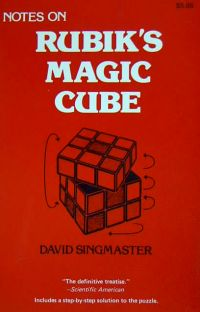My brother Joel gave me this classic book NOTES ON RUBIK'S MAGIC CUBE by David Singmaster, 1981, ISBN 0-89490-043-9. The three lines of small white text at the bottom say "The definitive treatise." -- Scientific American Includes a step-by-step solution to the puzzle.A cube on a keychain. When you do this cube, it helps to do the face with keychain first, and then try to keep the corner with the keychain at LBD.A Rubik's Mini-Cube, billed as "The Not-So-Little Challenge". You can solve it using the same moves as the moves for placing corners on a Rubik's Cube.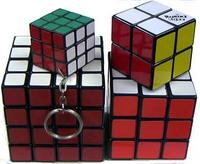A little cube family. Junior has a piercing.

The 4x4 Rubik's Revenge is about 2.6 inches (6.5 cm) wide.
The 3x3 Rubik's Cube is about 2.2 inches (5.6 cm) wide.
The 2x2 Rubik's Mini-Cube is about 1.5 inches (3.9 cm) wide.
The 3x3 keychain cube is about 1.2 inches (2.9 cm) wide.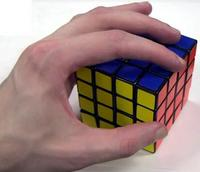To move pieces around quickly, it helps to have long fingers. In my younger days, I used to do 60 chins every day from a bar on a climber. I think that the daily stretch on my wrists helped to keep me from having repetitive stress carpal tunnel problems from cubing and from working as a computer programmer. One of the fastest cubists is a rock climber http://csel.cs.colorado.edu/~knightsd/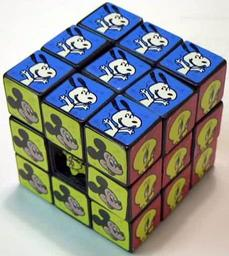I bought this cartoon cube in Buenos Aires from a street vendor in the park in Recoleta. I suspect that the maker of the cube did not bother to license the cartoon characters. This cube was not glued together well. If you look closely, you can see that Mickey Mouse's head popped off on one of the centers. Cubes with pictures are harder to solve than regular cubes because you must also rotate the centers correctly.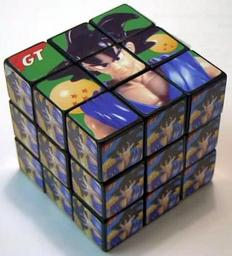I bought this Dragonball Z http://www.dragonballz.com/ cube from a street vendor in Recoleta for \$1 during a later trip to Buenos Aires in February, 2001 ../../200102buenosaires.html. This cube is tricky to solve because the faces look almost alike (except for the top face).I bought this PokeMon http://www.pokemon.com/ cube in Krakow, Poland, at a stand on the Rynek (market square) for 4 zloty (about \$1.03) on November 27, 2003 ../../poland/poland.html#cube. The front of the package says "OLD BRAND MAGIC SQUARE" up the left side, "New" inside a star at the upper right, and "NO. 8999 WARNING: CHOKING HAZARD - Small parts Not for children under 3 years MADE IN CHINA" across the bottom.The back has a six-step top-middle-bottom solution with small diagrams and text in Chinese and English, but the writing is hard to read because the letters are small and partially rubbed off and because a Polish "male elementy" small parts safety warning and a bar code sticker cover the first three steps.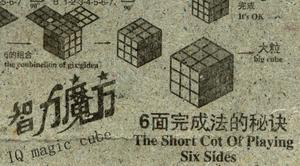The lower right of the back has some interesting text... The upper left of this picture has a diagram of a cube with the text "the conbinelion of gix gidea". The upper right has the text "It's OK" after the sixth step of the solution. The large cube below it says "big cube". The bold text below that says "The Short Cot Of Playing Six Sides" (with "Cot" for "Cut"). The diagonal text at the lower left says "IQ magic cube".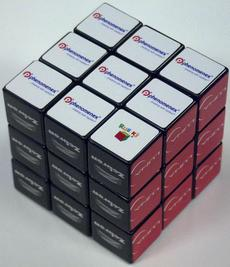I filled in a pharmaceutical crossword puzzle in a Phenomenex http://www.phenomenex.com/ catalog on February 18, 2004, to win this cube on April 10, 2004. The sides list the names of their products: white = phenomenex ...breaking with tradition, black = Zebron, red = explore LUNA, green = Jupiter Proteo, EZ:faast, Jupiter 300, and BioSep, yellow = strata, blue = synergi. This cube is an official Rubik's Cube (made in China) and moves very cleanly. It would be a good racing cube if it had normal colors. It came addressed to "Rusty Terrier at Scientific Programmers". Rusty was a dog ../../wondertwins.html#dogcicles. I use her name when I don't want to get on a mailing list. Rusty gets lots of mail even though she has been gone since 1985.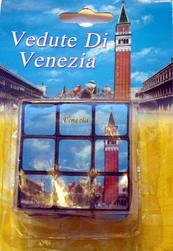Cube from Venice (January 2008).

See Joshua's Virtual Puzzle Museum http://www.twistypuzzles.com/ for a large selection of specialty cubes.The circles join in the solved position of this flat Rubik's Magic puzzle. The puzzle has a 1986 copyright by Rubik for the artwork and has an E. Rubik signature printed on the bottom. Matchbox made the puzzle in China. The puzzle has a few lengths of clear fishing line that hold the pieces together. The ends of the line are joined with metal clips. As you move the puzzle, the line (and the clips along with it) shifts position. Once a clip shifts between two pieces, the line becomes stuck, and the puzzle no longer works.

Christian Eggermont's page http://web.inter.nl.net/users/C.Eggermont/Puzzels/Magic/ shows other shapes but includes the warning: "To make the cube you have to be very careful since you probably stretch some of the wires trying to make the cube... which is not smart."
Jaap's puzzle page http://www.geocities.com/jaapsch/puzzles/magic.htm explains how the looped cords work to hold the puzzle together. TwistyPuzzles http://twistypuzzles.com/articles/magic-restringing.shtml has restringing instructions.The back of the puzzle has closed links.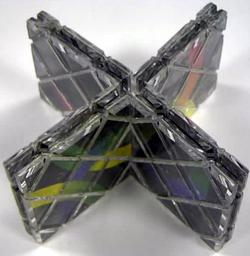The puzzle in a star configuration. A few of the clips have jammed. This is one of the few shapes that the puzzle can still form.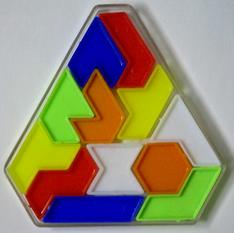My mother bought this tangram puzzle in March 2004 to give to one of her grandchildren (i.e. my cousins). She took it apart to test it and couldn't get it back together. I played with it for a few hours with no more success. I was already considering writing a program to solve it. We returned from visiting my brother Joel on April 6, 2004. I was exhausted that night and played with the puzzle at 3 am before going to bed, and somehow by luck, the pieces fell into place and I solved it in a few minutes. I took this picture.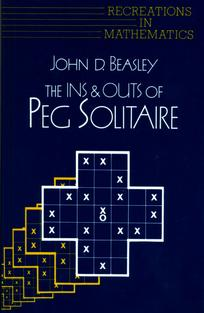My copy of "The Ins & Outs of Peg Solitaire" by John D. Beasley, 1985, ISBN 0-19-853203-2. This book is one of the best references for peg solitaire.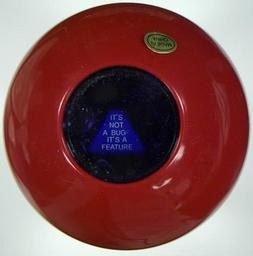A CDW http://www.cdw.com Magic 8-Ball for programmers showing the answer "IT'S NOT A BUG - IT'S A FEATURE". The bottom of the ball says "For your most commonly asked IT requests."

Here are the answers. I think that I have told people all of these, except the ones that are ads for CDW.
CDW HAS YOUR COMPUTING SOLUTIONS
I CAN'T TEST EVERYTHING
IT WORKED YESTERDAY
IT WORKS LIKE I PROGRAMMED IT
IT WORKS ON MY MACHINE
IT'LL BE FIXED IN THE NEXT RELEASE
IT'S A BETA - WHAT DID YOU EXPECT
IT'S AN UNLIKELY COINCIDENCE
IT'S JUST AN ISOLATED INCIDENT
IT'S NOT A BUG - IT'S A FEATURE
IT'S NOT SUPPOSED TO DO THAT
LOG ON TO WWW.CDW.COM
PLEASE SUBMIT A FORMAL REQUEST
PLUG IT IN
PROGRAM WORKS - MUST BE USER ERROR
REBOOT
SOMEONE CHANGED MY CODE
YOUR CDW ACCOUNT MANAGER CAN HELP

Other people use balls like this http://www.ericmackonline.com/ica/blogs/emonline.nsf/dx/how-does-he-answer-so-quickly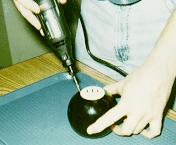The Unofficial Magic 8-Ball Home Page http://8ball.ofb.net/ demonstrates the dissection of an 8-ball in full color.